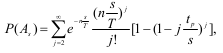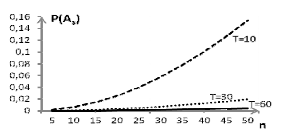# Series summation to infinite

Dear expert,

I’m trying to run a series summation in ROOT, regarding the probability of collisions in data transmission. I want to reproduce the following the graph attached, which is the result of the equation attached. There, n is the number of sensors transmitting, T is the repetition time, s is the observation time and tp is the transmission time…j is the number of transmissions within s time. I tried defining a function for this:

Double_t ProbCollision_St(Double_t n, Double_t s, Double_t t, Double_t T)
{
Double_t A = 0, B = 0, C = 0;
Double_t p = 0; Double_t Sum = 0;
Double_t kmax = 10000;

``````for(Double_t k = 2; k <= kmax; k++)
{
A = Exp(-n*s/T);
B = -Power(n*s/T,k);
C = 1 - Power((1 - k*t/s),k);
p = A*B*C/Factorial(k);
Sum += p;
}
return Sum;
``````

}

And then calling it inside a for statement:

void Collision_Statistics()
{
const Double_t tp = 3.2e-5;
const Double_t S = 180;
const Double_t T1 = 10, T2 = 30, T3 = 60;
const UInt_t stepN = 5;
const UInt_t maxN = 10;

``````Double_t N = {0};
Double_t P1_t = {0}; Double_t P2_t = {0}; Double_t P3_t = {0};
Double_t P1_s = {0}; Double_t P2_s = {0}; Double_t P3_s = {0};

for(UInt_t i = 1; i <= maxN; i++)
{
N[i-1] = i*stepN;
//cout << N[i-1] << endl;

P1_t[i-1] = ProbCollision_tp(N[i-1],tp,T1);
P2_t[i-1] = ProbCollision_tp(N[i-1],tp,T2);
P3_t[i-1] = ProbCollision_tp(N[i-1],tp,T3);

[b] P1_s[i-1] = ProbCollision_St(N[i-1],tp,S,T1);
P2_s[i-1] = ProbCollision_St(N[i-1],tp,S,T2);
P3_s[i-1] = ProbCollision_St(N[i-1],tp,S,T3);[/b]

cout<<scientific<<P1_s[i-1]<<endl;
}
``````

}

I’m printing and plotting the results, but everything seem to go to Zero…is there another way to set a summation like that?
ps: this is a Poisson Process